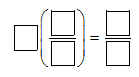# Fractions / Multiplying Fractions by a Whole Number

Activity 9 of 15
This lesson can be used to develop multiplication of a fraction by a whole number.

## Planning and Resources

Objectives
Students understand that the product of a whole number n and a fraction (a/b) is (na/b).

Vocabulary
commutative property of multiplication

Standard:

## Lesson Snapshot

#### Understanding

Have students explain how the visualization on the top number line is related to the value of the product; 6 x (1/5) can be seen as "6 copies of the unit fraction (1/5)"

### What to look for

Have students work together using the interactive number line to find the product of two numbers, then have them find an equivalent fraction for the product.

### Sample Assessment

Insert values into the blanks to make a true statement

(a number may be used more than once).

3, 5, 6, 8, 2, 10, 12